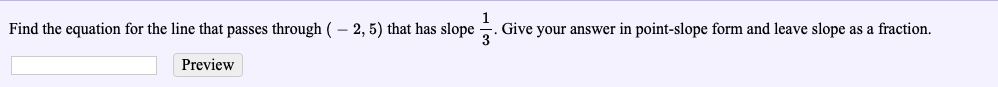# Find the equation for the line that passes through ( -2,5) that has slope . Give your answer in point-slope form and leave slope as a fraction.Preview

Question
3 viewshelp_outlineImage TranscriptioncloseFind the equation for the line that passes through ( -2,5) that has slope . Give your answer in point-slope form and leave slope as a fraction. Preview fullscreen
check_circle

Step 1

Point –slope formula is a general formula to obtain the line of the equation, while the slope of the line and a point on the line is given.

Let m is the slope of the line and (x1,y1) is appoint on the line. Then,

Step 2

Given that, the slope of the line is 1/3 and the line passes through the point (-2,5). Therefore,

Step 3

Substitute the values of m, x1 and x2 in the poin...

### Want to see the full answer?

See Solution

#### Want to see this answer and more?

Solutions are written by subject experts who are available 24/7. Questions are typically answered within 1 hour.*

See Solution
*Response times may vary by subject and question.
Tagged in

### Algebra